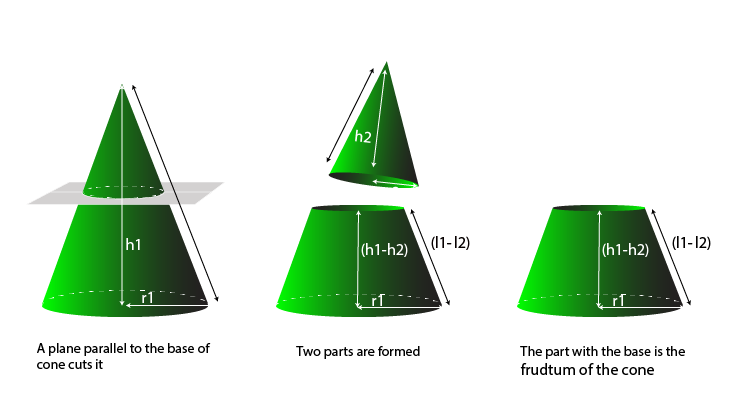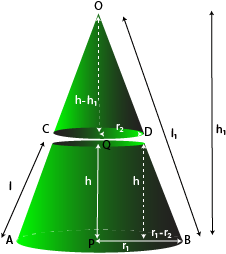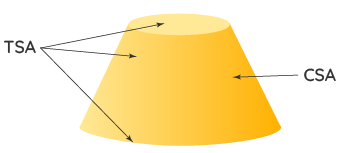Surface area and the volume of a frustum of a cone

## Objective

To obtain formulae for the surface area and the volume of a frustum of a cone

## What is a Frustum of Cone?

When we slice (or cut) a cone with a plane parallel to its base and remove the cone that is formed on one side of that plane, the part that is now left over on the other side of the plane is called a frustum of the cone.## Surface Area of Frustum of Cone

• The larger cone is considered as cone 1 and the smaller cone as cone 2.
• Let us consider h1 as the height, l1 as the slant height, and r1 as the radius of the larger cone 1. And consider h2 as the height, l2 as the slant height, and r2 as the radius of the smaller cone 2.
• The surface area will also be the difference of the surface areas of both the cones.
• Curved surface area of right circular cone 1 = πr1l1
• Curved surface area of right circular cone 2 = πr2l2
• Curved surface area of the frustum of cone = πr1l1 – πr2l2## Volume of Frustum Cone

• The larger cone is considered as cone 1 and the smaller cone as cone 2.
• Let us consider h1 as the height, l1 as the slant height, and r1 as the radius of the larger cone 1. And consider h2 as the height, l2 as the slant height, and r2 as the radius of the smaller cone 2.
• The height of frustum be H and its slant height is L.
• Now, the volume of the frustum of a cone = Volume of right circular cone 1 – Volume of smaller cone 2.
• The volume of right circular larger cone 1 = ⅓ π*r1h1
• Volume of right circular smaller cone 2 = ⅓ π*r2h2
• Therefore, the volume of the frustum of a cone V = ⅓ π*r1h1 - ⅓ π*r2h2 = ⅓ π(r1h1 - r2h2)## Surface Area of Frustum :• The surface area of a frustum is basically the sum of areas of its faces and is measured in square units like the area of any other shape. i.e., it is measured in cm2, m2, in2, etc.
• There are two types of surface areas with respect to the frustum.
• Curved Surface Area (CSA) for the frustum of a cone [OR] Lateral Surface Area (LSA) for the frustum of a pyramid.
• Total Surface Area (TSA) for the frustum of a cone (or) the frustum of a pyramid.

## Angle-Angle Similarity

• Two triangles are similiar, if their corresponding angles are equalTheir corresponding sides are in the same ratio (or proportion)
• Note that if corresponding angles of two triangles are equal, then they are known as equiangular triangles.
• The ratio of any two corresponding sides in two equiangular triangles is always the same.